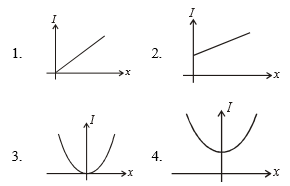Concept Questions :-

Moment of inertia
High Yielding Test Series + Question Bank - NEET 2020

Difficulty Level:

A particle is rotating under the central force, in which one of the following quantities is constant:

1.  torque

2.  angular momentum

3.  linear momentum

4.  none of these

Concept Questions :-

Angular momentum
High Yielding Test Series + Question Bank - NEET 2020

Difficulty Level:

A small object of uniform density rolls up a curved surface with an initial velocity v. It is reached upto a maximum height of $\frac{3{\mathrm{v}}^{2}}{4\mathrm{g}}$ w.r.t. the initial position. The object is: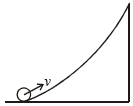1.  Ring

2.  Solid sphere

3.  Hollow sphere

4.  Disc

Concept Questions :-

Rolling motion
High Yielding Test Series + Question Bank - NEET 2020

Difficulty Level:

A point is at (x, y, z). What is its moment of inertia about x-axis: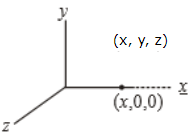1.  $\mathrm{m}\left({\mathrm{x}}^{2}+{\mathrm{y}}^{2}+{\mathrm{z}}^{2}\right)$

2.  $\mathrm{m}\left({\mathrm{x}}^{2}+{\mathrm{y}}^{2}\right)$

3.  $\mathrm{m}\left({\mathrm{y}}^{2}+{\mathrm{z}}^{2}\right)$

4.  $\mathrm{m}\left({\mathrm{x}}^{2}+{\mathrm{z}}^{2}\right)$

Concept Questions :-

Moment of inertia
High Yielding Test Series + Question Bank - NEET 2020

Difficulty Level:

What minimum friction coefficient is required for hollow sphere rolling without slipping on the inclined plane with an angle of inclination 37$°$ ?

1.  $\frac{3}{10}$

2.  $\frac{3}{14}$

3.  $\frac{3}{16}$

4.  None of these

Concept Questions :-

Rolling motion
High Yielding Test Series + Question Bank - NEET 2020

Difficulty Level:

The disc is placed on the rough horizontal surface given only angular velocity ${\omega }_{0}$. The velocity of the centre of mass when it starts pure rolling is:

1.  $\frac{{\omega }_{0}R}{2}$

2.  $\frac{{\omega }_{0}R}{3}$

3.  $\frac{{\omega }_{0}R}{4}$

4.  $\frac{{\omega }_{0}R}{6}$

Concept Questions :-

Rolling motion
High Yielding Test Series + Question Bank - NEET 2020

Difficulty Level:

A force 2N is along line y = 3x + 4. The torque about the origin is:

1.  $\frac{5}{\sqrt{10}}\mathrm{Nm}$

2.  $\frac{7}{\sqrt{10}}\mathrm{Nm}$

3.  $\frac{6}{\sqrt{10}}\mathrm{Nm}$

4.  $\frac{8}{\sqrt{10}}\mathrm{Nm}$

Concept Questions :-

Torque
High Yielding Test Series + Question Bank - NEET 2020

Difficulty Level:

A uniform bar of length 6a and mass 8m lies on a smooth horizontal table. Two-point
masses m and 2m moving in the same horizontal plane with speeds 2v and v
respectively strike the bar as shown below in the figure and stick to the bar after the collision. Denoting angular velocity about the center of mass, total energy, and center of mass velocity of $\omega$, E and ${\mathrm{v}}_{\mathrm{c}}$ respectively, we have after collision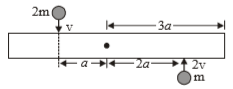1.

2.

3.

4.

Concept Questions :-

Angular momentum
High Yielding Test Series + Question Bank - NEET 2020

Difficulty Level:

A stone is projected from a horizontal plane. It attains maximum height 'H' & strikes a stationary smooth wall & falls on the ground vertically below the maximum height. Assume the collision to be elastic, the height of the point on the wall where ball will strike is: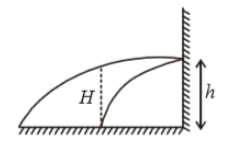(1) $\frac{H}{2}$

(2) $\frac{H}{4}$

(3) $\frac{3H}{4}$

(4) None of these

Concept Questions :-

Linear momentum
High Yielding Test Series + Question Bank - NEET 2020

Difficulty Level:

A train of mass M is moving on a circular track of radius 'R' with constant speed v. The length of the train is half of the perimeter of the track. The linear momentum of the train will be

1. 0

2. $\frac{2\mathrm{Mv}}{\mathrm{\pi }}$

3. MvR

4. Mv

Concept Questions :-

Linear momentum